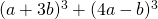Chapter 7: Factoring

# 7.6 Factoring Quadratics of Increasing Difficulty

Factoring equations that are more difficult involves factoring equations and then checking the answers to see if they can be factored again.

Example 7.6.1

Factor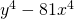.

This is a standard difference of squares that can be rewritten as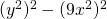, which factors to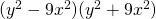. This is not completely factored yet, since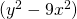can be factored once more to give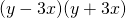.

Therefore,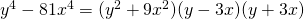.

This multiple factoring of an equation is also common in mixing differences of squares with differences of cubes.

Example 7.6.2

Factor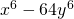.This is a standard difference of squares that can be rewritten as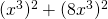, which factors to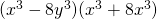. This is not completely factored yet, since both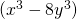and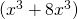can be factored again.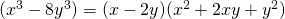and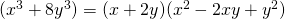This means that the complete factorization for this is: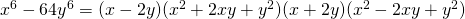Example 7.6.3

A more challenging equation to factor looks like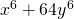. This is not an equation that can be put in the factorable form of a difference of squares. However, it can be put in the form of a sum of cubes.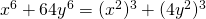In this form,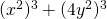factors to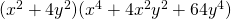.

Therefore,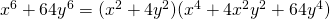.

Example 7.6.4

Consider encountering a sum and difference of squares question. These can be factored as follows: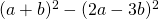factors as a standard difference of squares as shown below: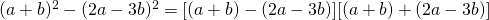Simplifying inside the brackets yields: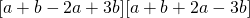Which reduces to: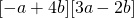Therefore: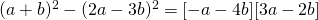Examples 7.6.5

Consider encountering the following difference of cubes question. This can be factored as follows: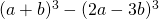factors as a standard difference of squares as shown below: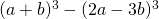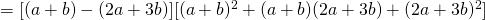Simplifying inside the brackets yields: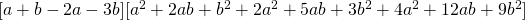Sorting and combining all similar terms yields: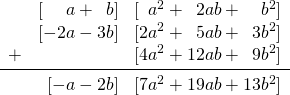Therefore, the result is: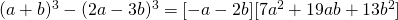# Questions

Completely factor the following equations.

1.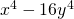2.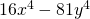3.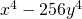4.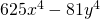5.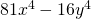6.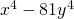7.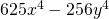8.9.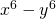10.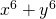11.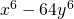12.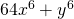13.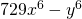14.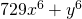15.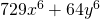16.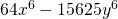17.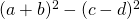18.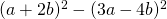19.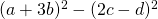20.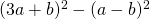21.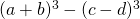22.